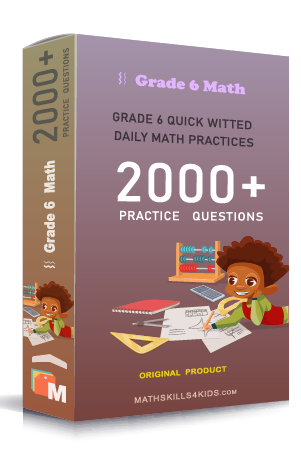# Grade 6 Multiplying and dividing decimals worksheets with solutions

Subject
Math

Resource Type
Worksheets, Printables, Homeschool

Standards
CCSS 6.NS.B.3

Format
PDF (17.9 MB | 28 pages → Exercises + Solutions)

• ### -15% OFF Over \$100

Promo Code: TOPLA

After Purchase

\$3.15

This package allows you to practice the following skills

Estimating product of decimal numbers

Multiplying decimals

Solving inequalities with decimals multiplication

Dividing decimals by whole numbers

Dividing decimals by whole numbers word problems

Dividing decimals by powers of ten

Solving division with a decimal quotient

Dividing decimal by decimal

This product is a part of the Mega Pack
* Grade 6 quick-witted daily math practices *